# Business Mathematics and Statistics Question Paper' 2021 (Held in 2022), Dibrugarh University B.Com 2nd Sem Non Hons

## 2021 (Held in January/February, 2022)COMMERCE (Discipline Specific Course)(For Non-Honours)Paper: CC-203 (Business Mathematics and Statistics)Full Marks: 80Pass Marks: 32Time: 3 hoursThe figures in the margin indicate full marks for the questions.

PART – A

(Marks: 32)

1. Write True or False: 1x3=3

1. If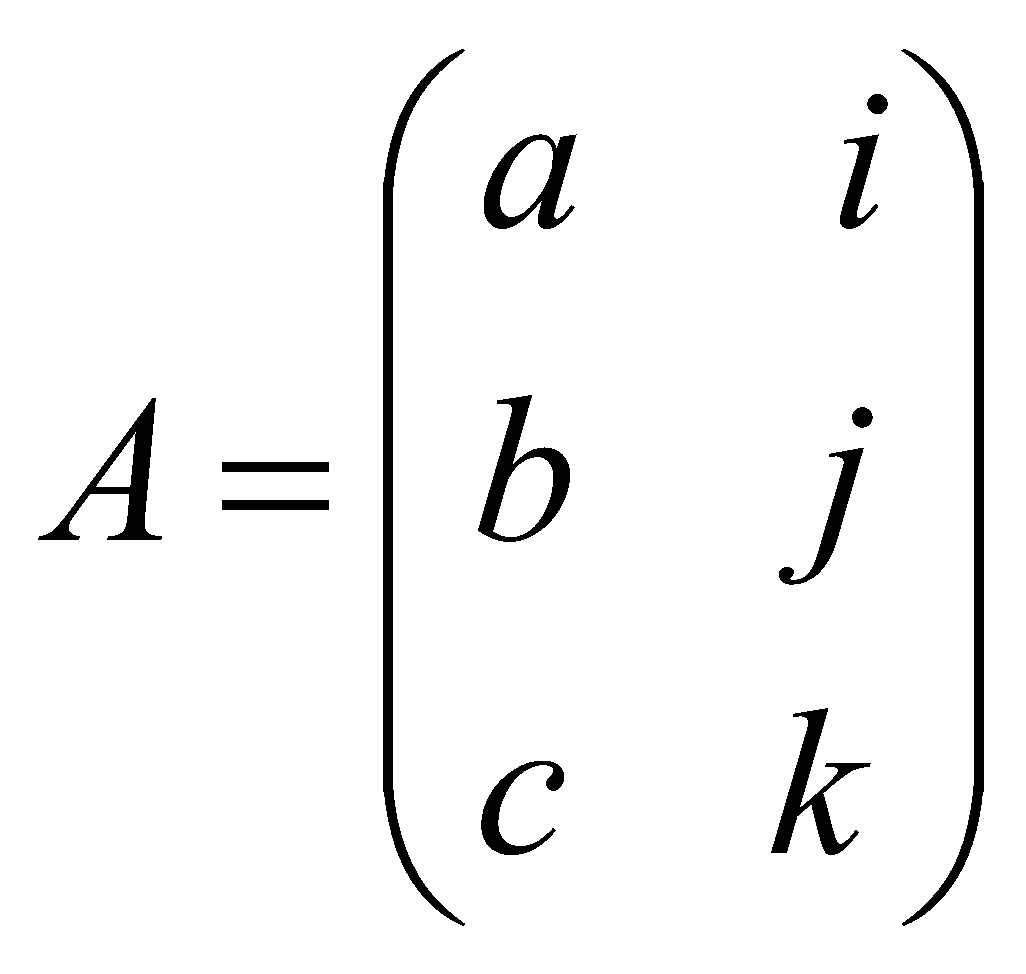, then the value of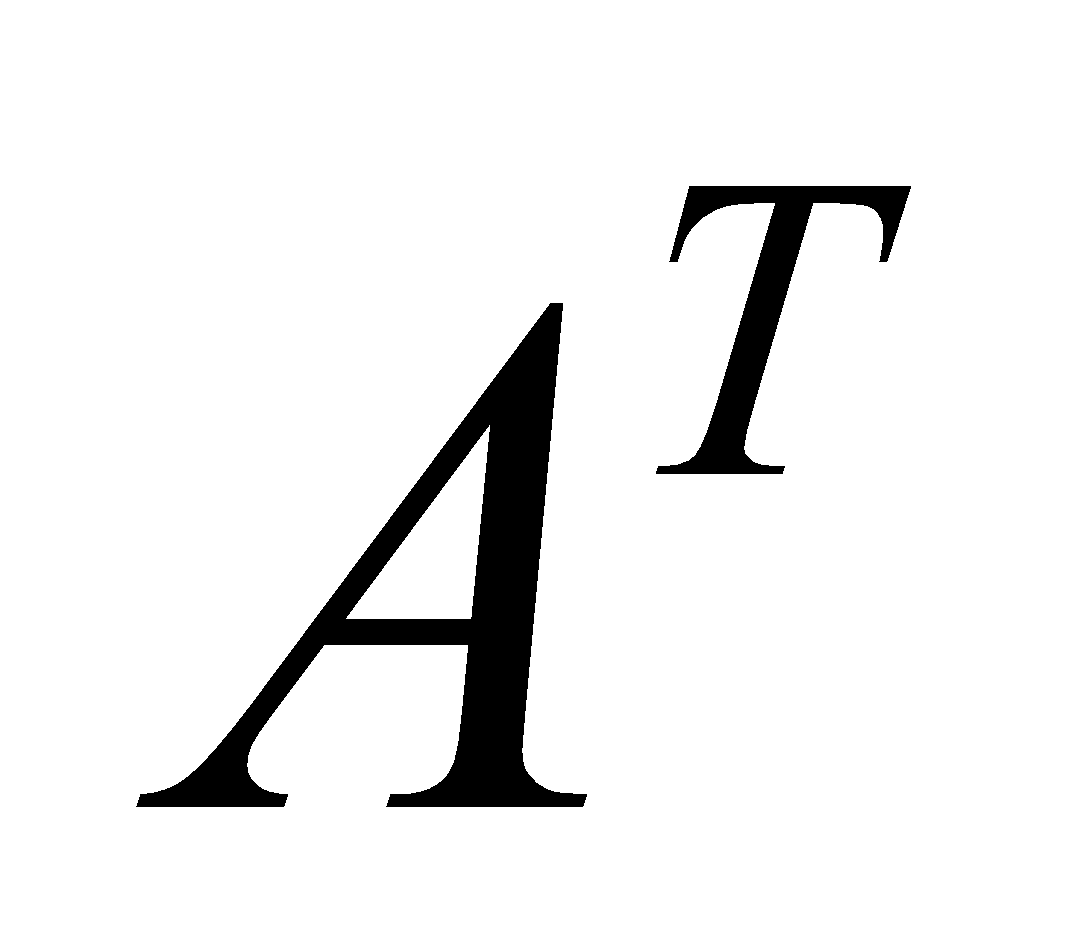is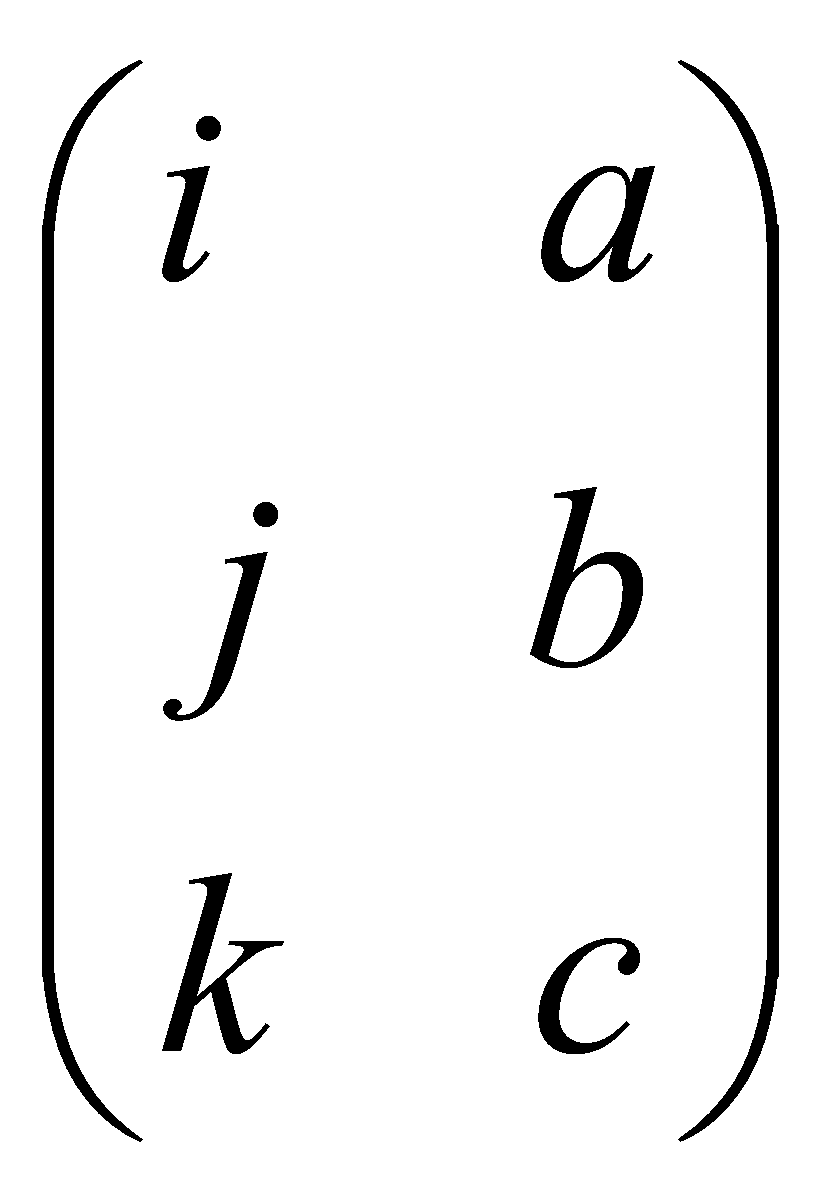.

2. Derivative of a constant is zero.

3. Simple interest on Rs. 500 at 4% p.a. for 30 months is Rs. 50.

2. If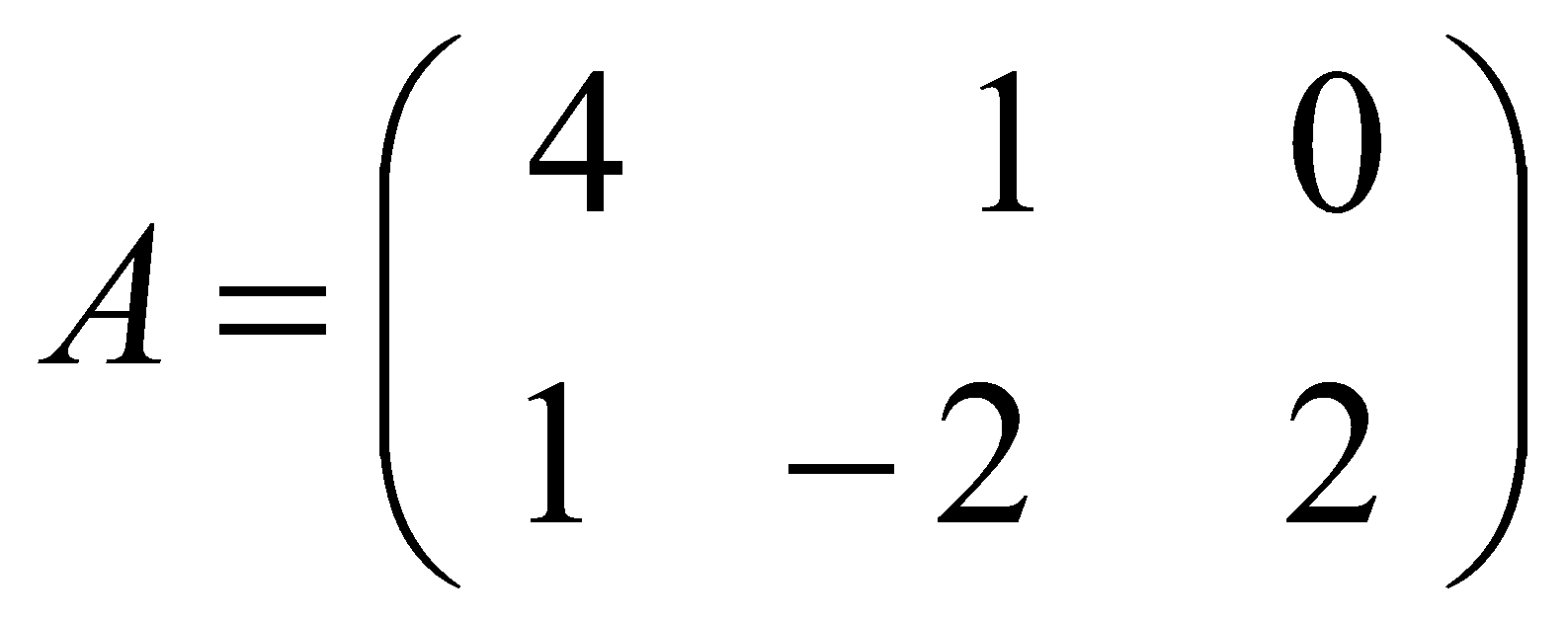,and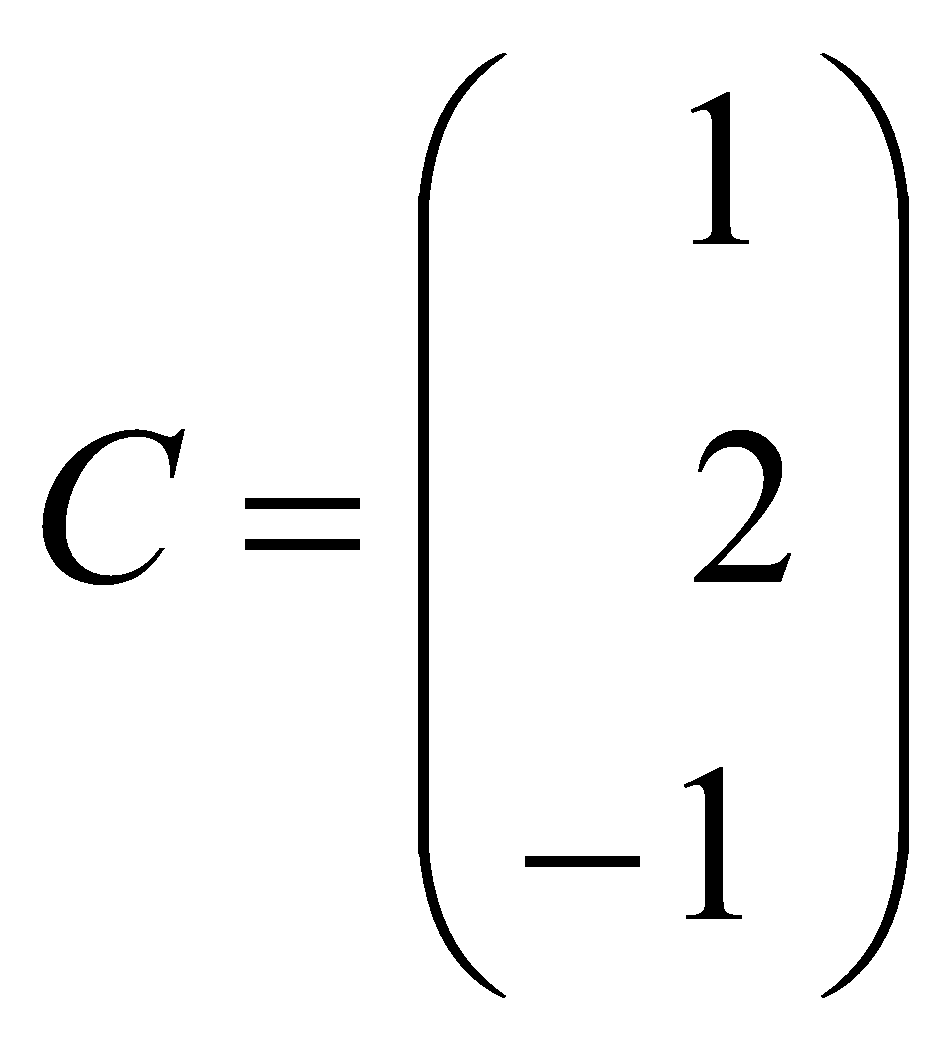, find matrix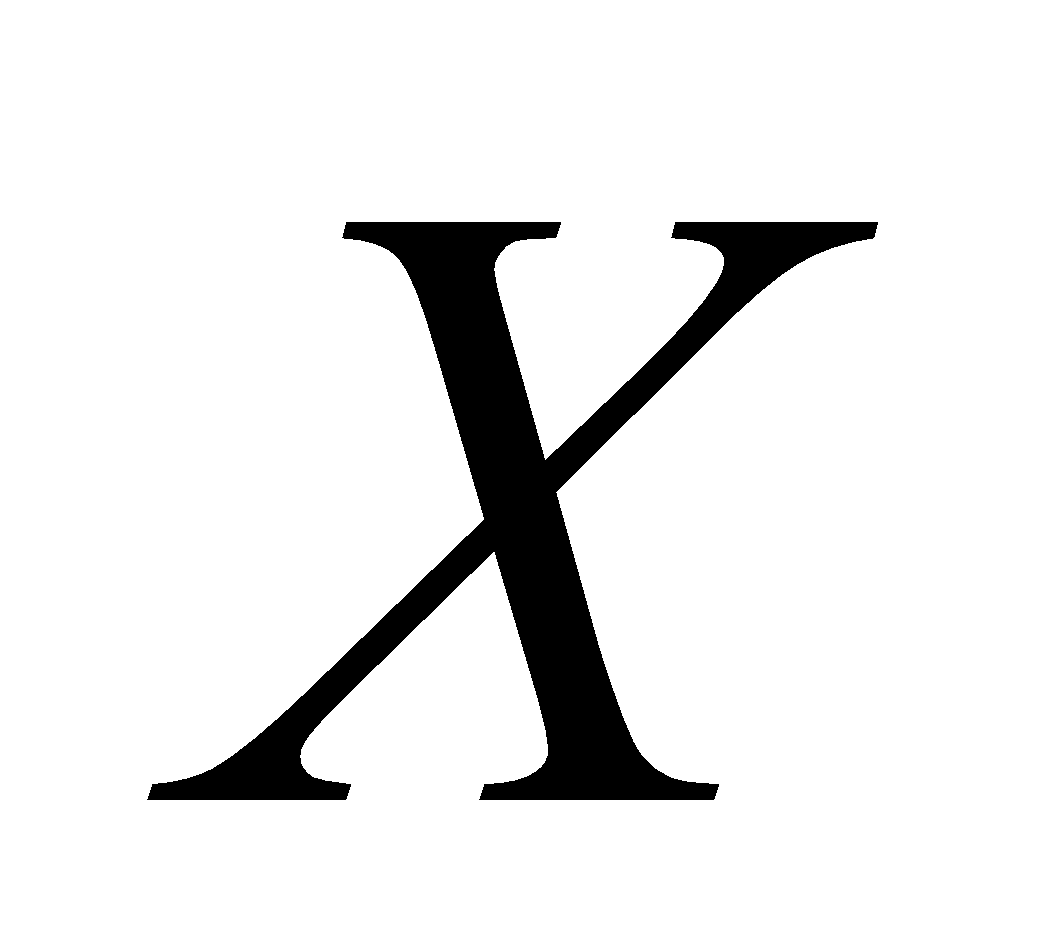such that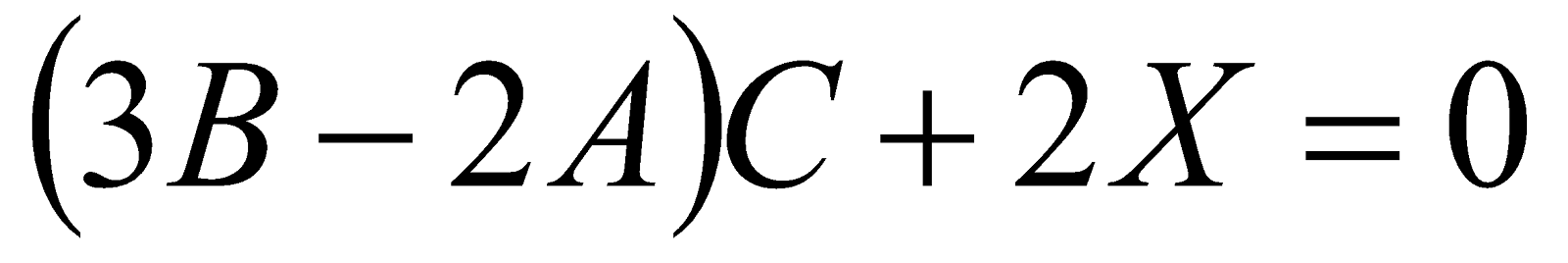.       3

3. If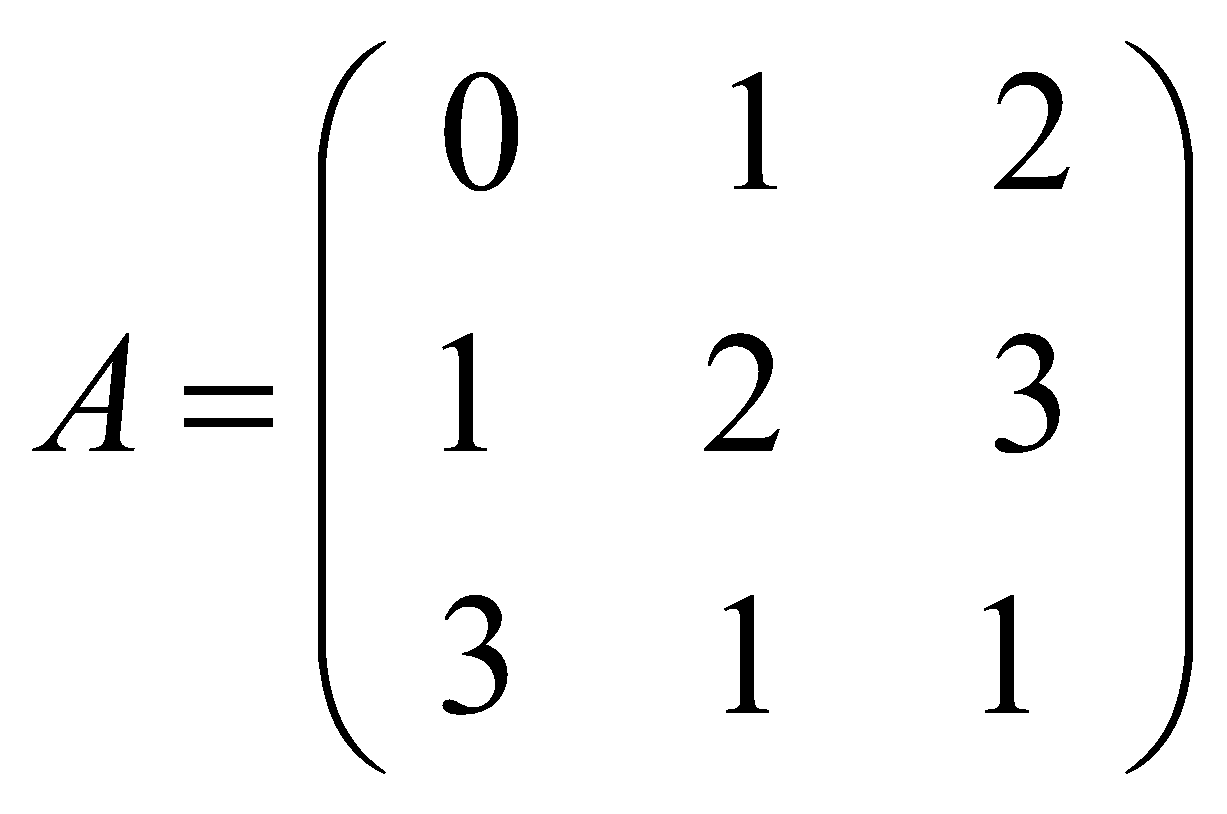, then find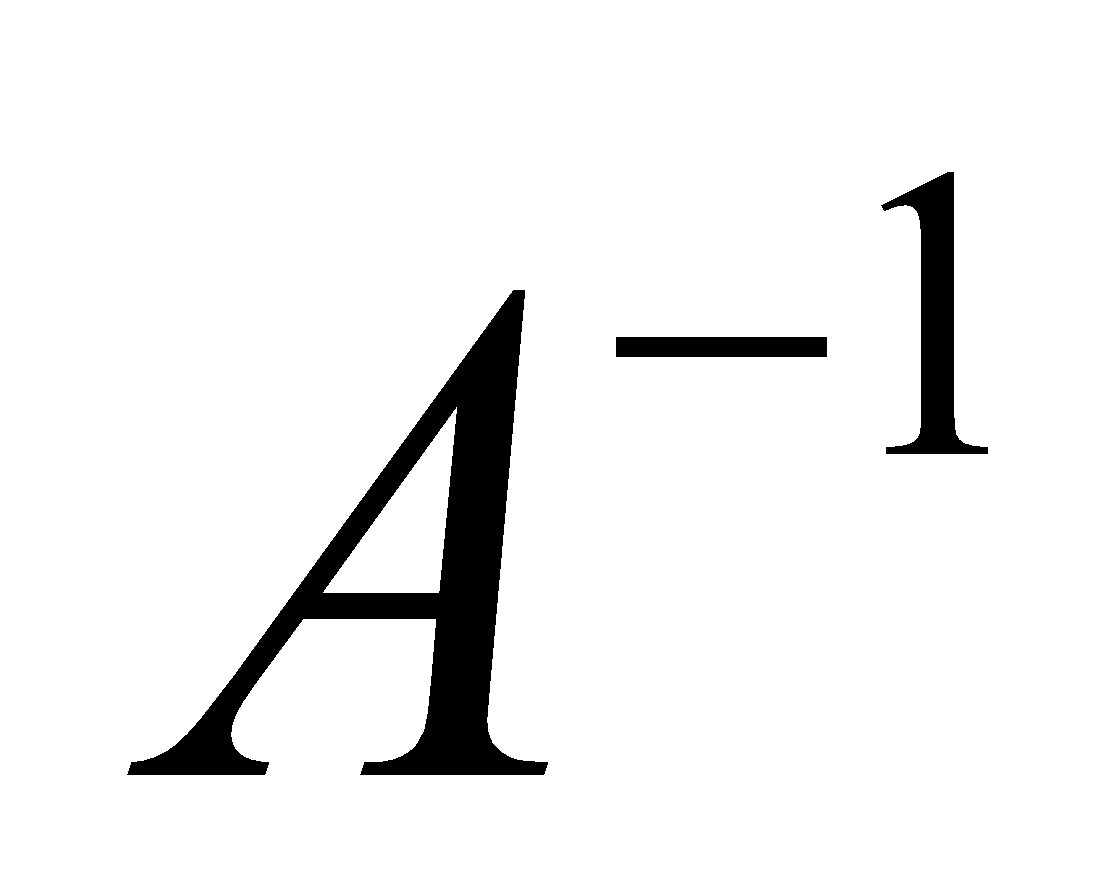. 6

Or

If, then prove that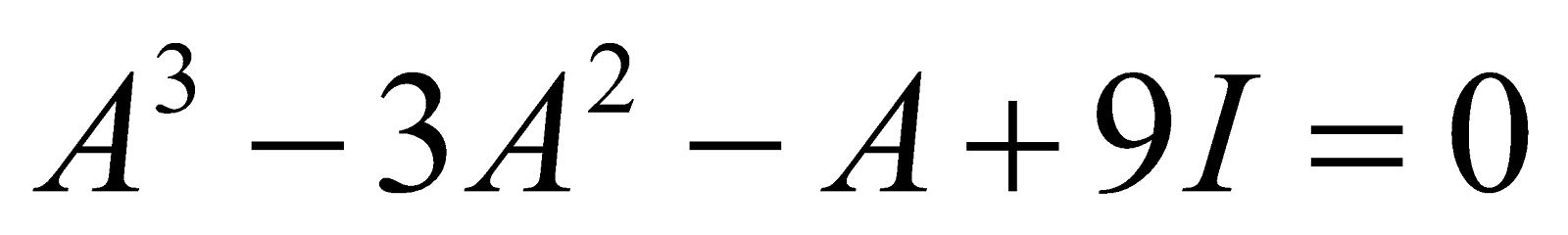4. Find the value of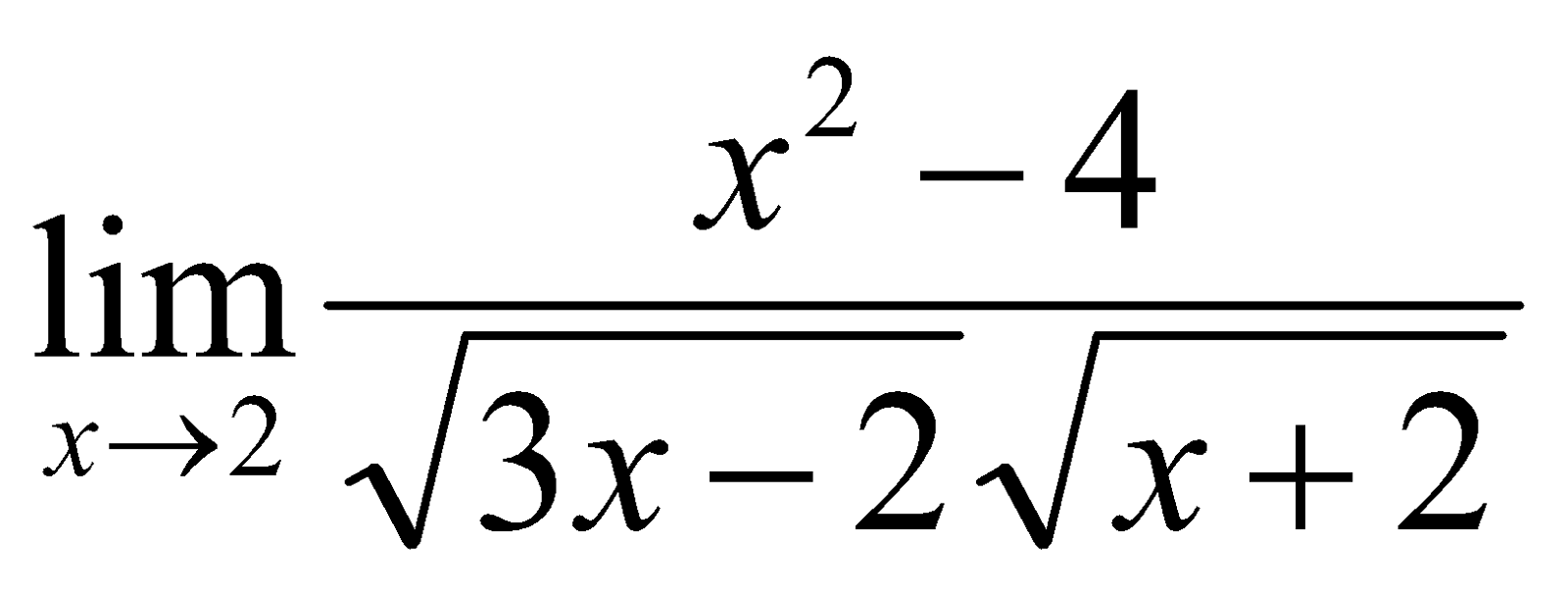. 3

5. Find, if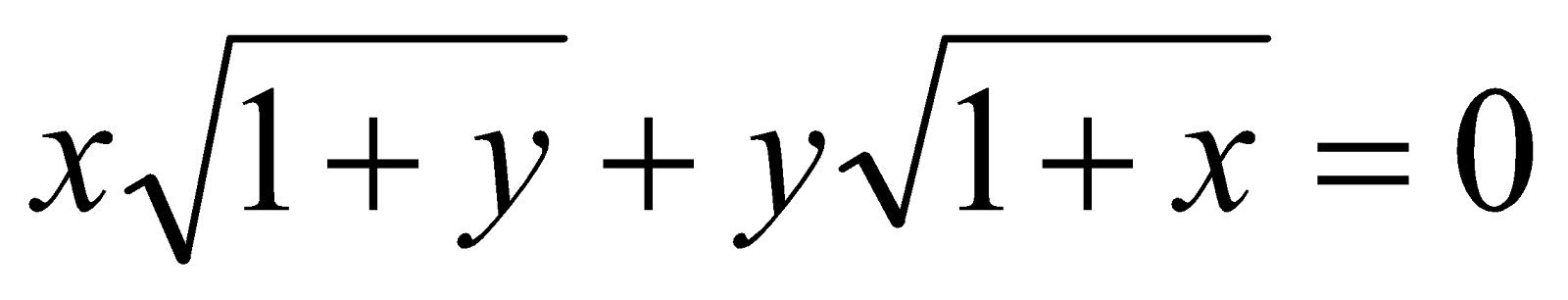. 6

Or

If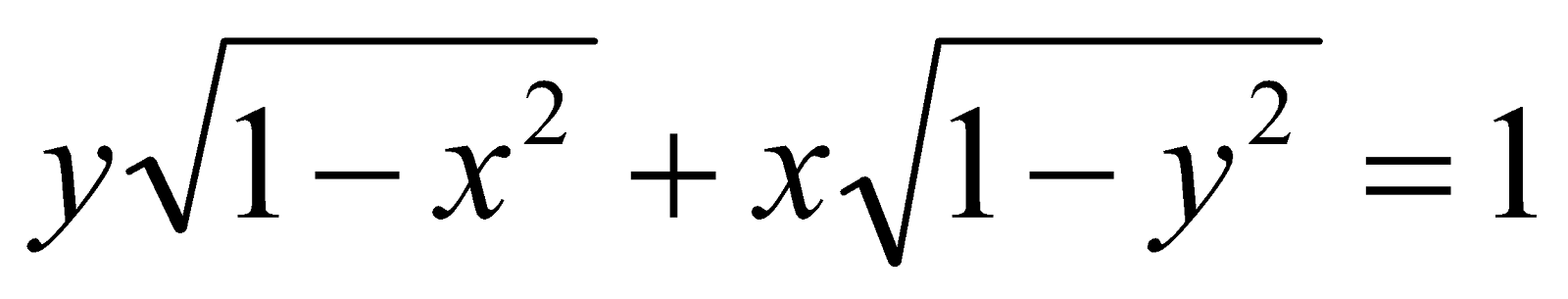, then prove that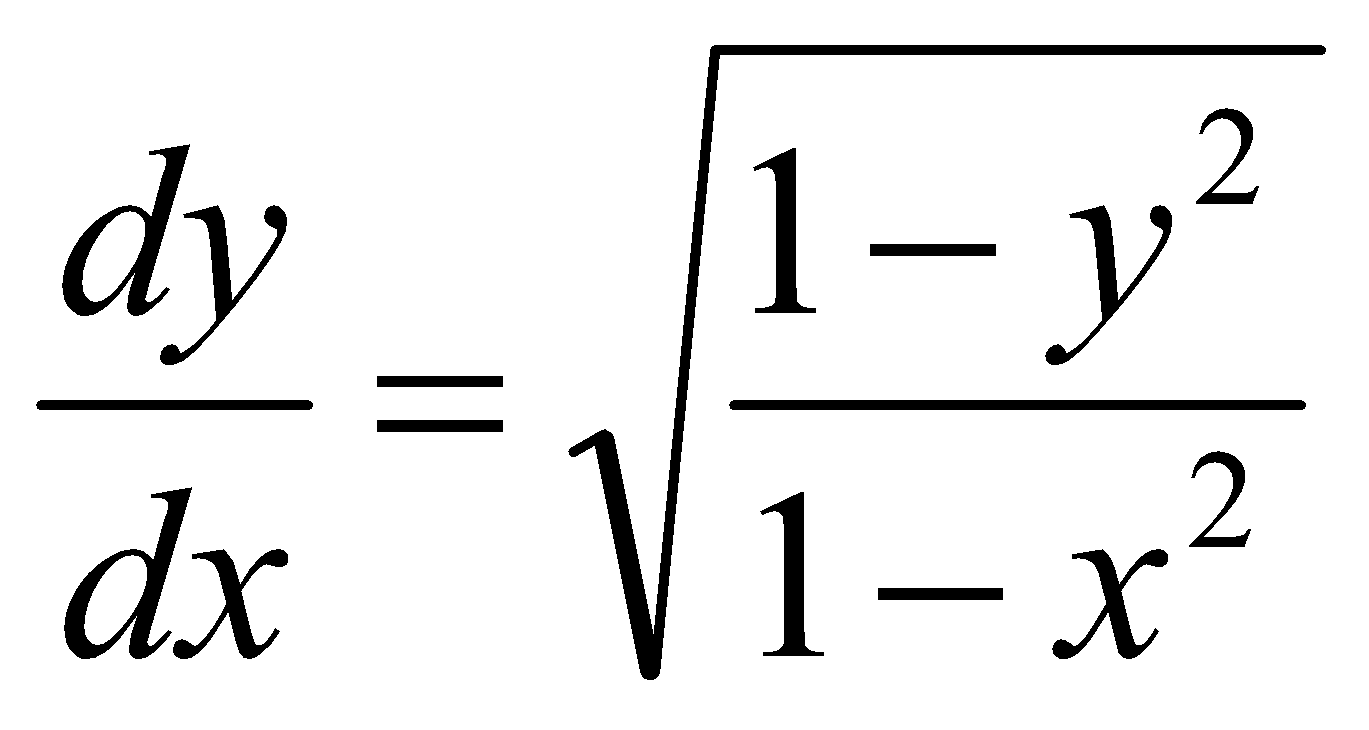6. Distinguish between nominal rate of interest and effective rate of interest with suitable example. 5

7. Compound interest on a sum for 2 years is Rs. 920.25. Simple interest on the sum for the same time is Rs. 900. Find the sum and rate of interest. 6

Or

Find the nominal rate of interest percent p.a. interest payable half-yearly which is equivalent to the effective rate of 4% p.a.

PART – B

8. Fill up the gaps: 1x5=5

1. ______ is regarded as the best measure of central tendency.

2. For a symmetrical distribution, values of mean, median and mode are ______.

3. ______ correlation deals with qualitative characteristic.

4. Correlation coefficient is the ______ of two regression coefficient.

5. The index number for the base year is taken as ______.

9. Answer either (a) or (b):

1. (1) In a moderately asymmetrical distribution, mode and mean are 32.1 and 35.4 respectively. Find the median.

(2) Which measure of variation is regarded as the best and why? 3+5

(3) Calculate the standard deviation for the following data: 6

 Wages (in Rs.) No. of man 0 and above20 and above 40 and above 60 and above 80 and above 100 and above 5045341660

Or

1. (1) Prove that for any two positive numbers,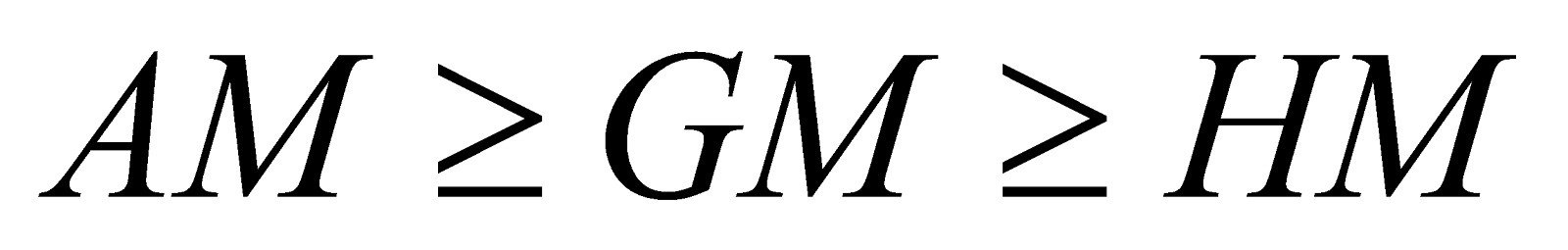. 3

(2) State the characteristics of a good measure of variation. 5

(3) The mean and standard deviation of 100 observations are 50 and 5, and that of another 150 observations are 40 and 6 respectively. Find the combined standard deviation of this 250 observations. 6

10. Answer either (a) or (b):

1. (1) What are the properties of the two regression coefficient? 3

(2) Prove that Karl Pearson’s coefficient of correlation is independent of change of origin and scale of measurement. 5

(3) Calculate the coefficient of correlation from the following data: 6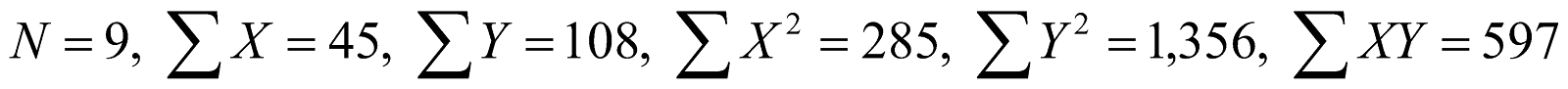Or

1. (1) Distinguish between Karl Pearson and Spearman correlation coefficient. 3

(2) Calculate the coefficient of rank correlation from the data given below: 5

 X: 48 33 40 9 16 65 24 18 44 20 Y: 13 10 24 6 15 4 20 9 10 19

(3) Derive the regression equation of X on Y from the following data: 6

 X: 35 42 20 50 72 64 Y: 40 48 24 60 84 68

11. Answer either (a) or (b):

1. (1) If the annual trend equation of a time series be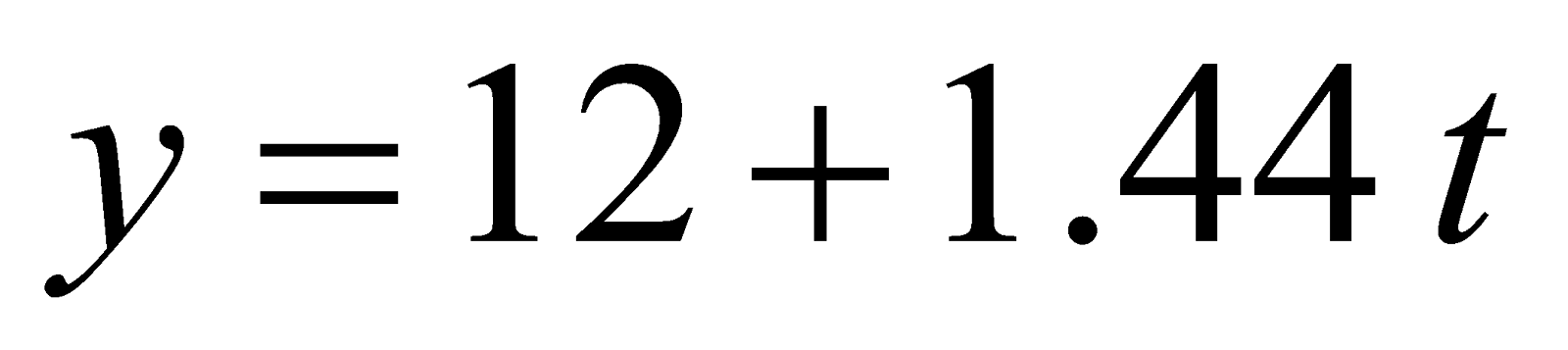(origin = 1990, t unit = 1 year), find the trend line equation with 1995 as origin. 3

(2) What are the uses of index number? 5

(3) Using Fisher’s formula calculate quantity index number from the data given below: 7

 Item 2017 2019 Price (in Rs.) Quantity Price (in Rs.) Quantity ABCDE 534117 50100603040 10461410 56120602436

Or

1. (1) What do you understand by secular trend? What are the factors responsible for trend in a time series?     3

(2) Estimate trend values by using 4-yearly moving average for the following data: 5

 Year Profit 20112012201320142015201620172018 6046535448484251

(3) Using the method of least square, calculate the trend values for the data given below: 7

 Year: 2001 2002 2003 2004 2005 2006 Sales: 60 72 75 65 80 85

***